• 使用CSS在宽高不确定的父元素内画一个正方形
需求：父元素宽高不确定，在内部画一个正方形
<div class="box">
<div class="inner"></div>
</div>

.box {
/* 为了方便演示：这里设置成固定宽高了 */
width: 200px;
height: 300px;
border: 1px solid red;
}

.inner {
/* 可以设置成100%或者其它的百分比 */
width: 50%;
background-color: #007fff;
}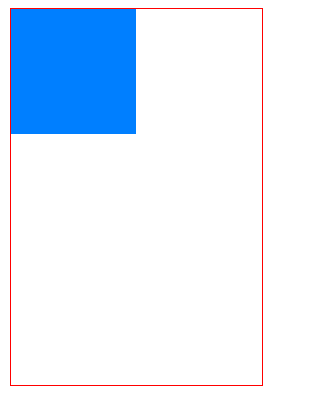原理：当padding 的取值是百分比的时候，它是相对于父元素的宽度； 一个div在没有明确设置高度，里边又没有内容的情况下，高度是0。所以我们可以在垂直方向上设置padding-top或者padding-bottom。注意设置padding-left或padding-right不会生效（因为子元素的高度依然是0）。

前端学习交流QQ群，群内学习讨论的氛围很好，大佬云集，期待您的加入：862748629 点击加入

展开全文css padding 前端
• 一、如何绘制一个任意大小的正方形：import turtle bob=turtle.Turtle() #turtle模块提供了一个叫作Turtle的函数，用于创建一个Turtle对象 bob._delay=1 def square(t,length): for i in range(4): t.fd(length) #...
一、如何绘制一个任意大小的正方形：
import turtle

def square(t, length):  #绘制一个正方形
t._delay = 10   #设置乌龟的爬行速度
for i in range(4):
t.fd(length)
t.lt(90)

def main():
bob=turtle.Turtle() #turtle模块提供了一个叫作Turtle的函数，用于创建一个Turtle对象
square(bob,100)
turtle.done()
return "Done!"

if __name__ =='__main__':
main()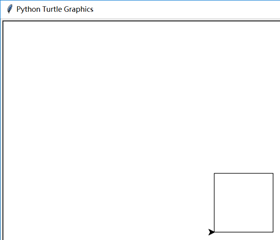二、如何绘制任意边数的多边形-进行一次泛化
import turtle

def polygon(t, n, length):  #绘制正多边形
t._delay = 10   #设置乌龟的爬行速度
angle = 360.0 / n
for i in range(n):
t.fd(length)
t.lt(angle)

def main():
bob=turtle.Turtle() #turtle模块提供了一个叫作Turtle的函数，用于创建一个Turtle对象
polygon(bob, n=10, length=30)
turtle.done()
return "Done!"

if __name__ =='__main__':
main()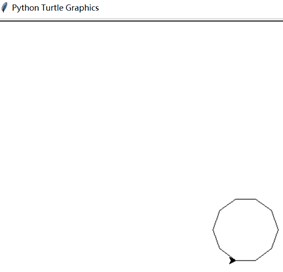三、如何绘制一个圆-再进行一次泛化

import turtle
import math

def polygon(t,n,length):   #绘制正多边形
angle=360.0/n   #角度可能不是整数
for i in range(n):
t.fd(length)
t.lt(angle)

def circle(t,r):        #用边数很多的正多边形来近似圆形
circumference = 2 * math.pi * r
n=int(circumference / 3)+1
length = circumference / n
polygon(t,n,length)
turtle.done()

def main():
bob=turtle.Turtle() #turtle模块提供了一个叫作Turtle的函数，用于创建一个Turtle对象
circle(bob,100)
return "Done!"

if __name__ =='__main__':
main()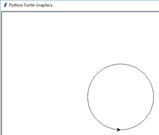四、如何绘制圆弧-再进行一次泛化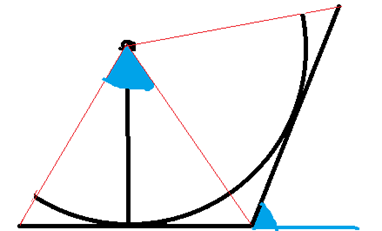上图为示意图，展示了如何用多边形去拟合圆弧，从几何上容易证明：
两个蓝颜色的角大小相等，该性质将在程序中用到

import turtle
import math

def polyline(t, n, length, angle):  #绘制多边线
t._delay = 10   #设置乌龟的爬行速度
for i in range(n):
t.fd(length)
t.lt(angle)

def arc(t,r,angle):
arc_length=2 * math.pi * r *angle / 360.0
n=int(arc_length / 3)+1

step_length=arc_length / n   #确定步长
step_angle = float(angle) / n  #确定每次的偏角
polyline(t, n, step_length, step_angle)

def main():
bob=turtle.Turtle() #turtle模块提供了一个叫作Turtle的函数，用于创建一个Turtle对象
arc(t=bob, r=30, angle=200)
turtle.done()
return "Done!"

if __name__ =='__main__':
main()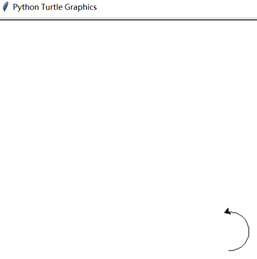展开全文多边形
• 给定一个整数，确定它是否是正方形数字： 在数学中，平方数或正方形是整数，它是整数的平方; 换句话说，它是一些整数与自身的产物。 例如： isSquare(-1) returns false isSquare(0) returns true isSquare(3) ...
给定一个整数，确定它是否是正方形数字：
在数学中，平方数或正方形是整数，它是整数的平方; 换句话说，它是一些整数与自身的产物。
例如：
isSquare(-1) returns false
isSquare(0) returns true
isSquare(3) returns false
isSquare(4) returns true
isSquare(25) returns true
isSquare(26) returns false
代码：
public class Square {
//方法一
public static boolean isSquare(int n) {
boolean flg=false;
double num=Math.sqrt(n);
int z=(int)num;
if(z==num){
flg=true;
}
return flg;
}

public static boolean isSquare1(int n) {
return Math.sqrt(n) % 1 == 0;
}

//方法二
public static void main(String[] args) {
System.out.println("num:"+isSquare(9));
System.out.println("num:"+isSquare1(9));
}
}
展开全文Java
正常情况下，要想设置一个正方形我们只需要设置特定的宽高即可
div {
width: 100px;
height: 100px;
}

div {
width: 20%;
background: red;
}

展开全文css css3
• C++用*和空格输出一个正方形及其对角线分析代码思路：下面分析外层for里面的两个部分：1. if 的要求是能输出正方形的首行和尾行，则判断可写为：2. else这里用来分隔中间几行代码的输入。 零基础起步程序猿，人生中...c++ 程序人生
• //先剪出一个正方形   Bitmap bitmap = Bitmap . createBitmap ( source , fromX , fromY , fSize , fSize ) ;   float scale = 1.0f * size / fSize ;   //设置x，y缩放比例   matrix . ...android
• 这里想做的是一个正方形的小抽屉柜，首先根据设计草图标出尺寸计算出所需四片木板的长度。其中，有两个边的木板是要靠着燕尾榫机的左边制作，另两个边的木板是要靠着燕尾榫机的右边制作。修出四片木板。试靠着看看，...
• 四枚硬币，摆成一个正方形，不知道硬币的正反面，然后我可以指定翻若干个硬币，对手可以随机的转动这个正方形。如果某一时刻，所有硬币朝向相同就算我赢了。问有没有必胜策略。 思路： 我们用1表示硬币正面，用0...
• Problem Description 根据读入的正方形的边长（边长为正整数），输出其正方形...每次读入一个边长，便输出其正方形的面积，每一行输出一个正方形的面积。 Sample Input 1 3 Sample Output 1 9 Hint Source 代码： im...
• 确定正方形的左上角后，根据左上角所在的行和列计算可能的最大正方形的边长（正方形的范围不能超出矩阵的行数和列数），在该边长范围内寻找只包含 11 的最大正方形； 每次在下方新增行以及在右方新增列，判断...java
• 我正在努力创建一个由均匀分布的正方形链组成的布局，这些正方形可以填充可用空间。使用Android Constraintlayout创建一排均匀分布的正方形我到底想要的布局，看起来像这样： 的想法是，与不同的宽高比手机这个...
• 一个由 0 和 1 组成的二维矩阵内，找到只包含 1 的最大正方形，并返回其面积。 示例: 输入: 1 0 1 0 0 1 0 1 1 1 1 1 1 1 1 1 0 0 1 0 输出: 4 思路 动态规划： 确定数组的意义。dp[i][j]表示以i，j为右下角的只...
•  * 功能：在二维平面上，有两个正方形，请找出条直线，能够将这两个正方形对半分。  * 假定正方形的上下两条边与x轴平行。  */ /** * 考虑： * 线的准确含义，可能性有： * 1）由斜率和y轴截距确定； * ...java 算法 数据结构 数学
• 用C#完成计算过程(无聊的人) 代码实现以迭代假设每像素点作为正方形左上角 以确定正方形个数 可运行的程序在./bin/debug/xxx.exe
• 依次类推，最后是边长为n的一共有1,就是最大的这个正方形所以一共有的正方形是：1^2+2^2+3^2+------+n^2令s=1^2+2^2+--------n^2.  其结果可猜测到是关于n的三次式,设s=an^3+bn^2+cn+d.分别取n=0,s=1；n=1,s=1...
• 593. 有效的正方形 给定二维空间中四点的坐标，返回四点是否可以构造一个正方形一个点的坐标（x，y）由一个有两个整数的......